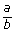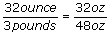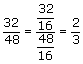## Definition Of Ratio

A ratio is a comparison of two numbers by division.

### Examples of Ratio

4 : 7, 1 : 6, 10 : 3 etc. are examples of ratio.
Any ratio a : b can also be written as ‘a to b’ or.

### Solved Example on Ratio

#### Ques: Write the ratio 32 ounce to 3 pounds in its simplest form.

##### Choices:

A. 2:3
B. 3:2
C. 4:2
D. 2:4
Step 1:[Convert pounds to ounces in order have matching units.]
Step 2:[Divide the numerator and the denominator by the G.C.F, 16.]